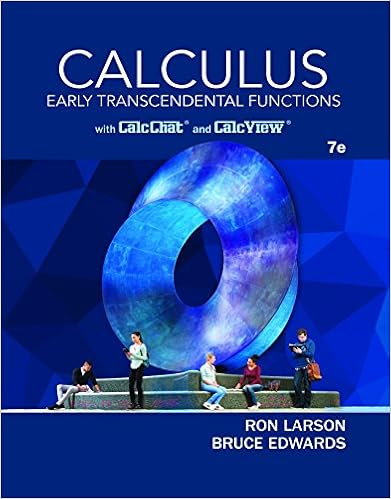# 2007Test3Solutions - MATH 2007A Test 3 Solutions November 9...

• Test Prep
• 2
• 100% (1) 1 out of 1 people found this document helpful

This preview shows page 1 - 2 out of 2 pages.

##### We have textbook solutions for you!
The document you are viewing contains questions related to this textbook.The document you are viewing contains questions related to this textbook.
Chapter 13 / Exercise 32
Calculus: Early Transcendental Functions
Edwards/LarsonExpert Verified
MATH 2007ATest 3 SolutionsNovember 9, 2017[Marks]1. Evaluate the improper integral or show that it diverges.
002. Consider the parametric curvex= 2t2+ 6t,y=t2+ 4t.(a) Finddydx.(b) Findd2ydx2.
3. Consider the parametric curvex= 2t,y= 2t3-3t2.(a) Finddydx.(b) Find the value(s) oftand the corresponding point(s) (x, y) where the tangent ishorizontal.(c) Find the equation of the tangent line att= 2.(d) Findd2ydx2.(e) Find the intervals oftwhere the graph is concave up and the intervals where itis concave down.
##### We have textbook solutions for you!
The document you are viewing contains questions related to this textbook.The document you are viewing contains questions related to this textbook.
Chapter 13 / Exercise 32
Calculus: Early Transcendental Functions
Edwards/LarsonExpert Verified
2
•••